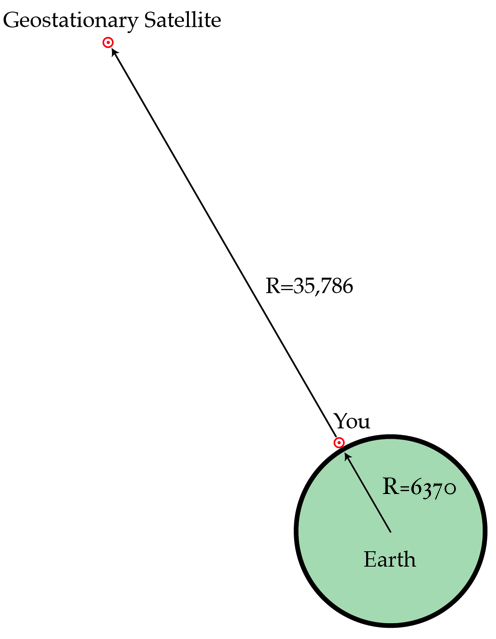Classical Mechanics

# Angular Kinematics - Problem SolvingA ceiling fan is switched off and, after 36 rotations, its angular velocity falls by half.

How many more rotations will it make before coming to rest?

Details and Assumptions

• The angular deceleration of the fan is uniform.

A mass $m = 2 \text{ kg}$ which is attached to a vertical rod by a string revolves around the rod on a frictionless horizontal surface. As the mass revolves, the string winds to the rod, causing the distance between the mass and rod to decrease. When the distance between the mass and the rod is $r_i = 12 \text{ m},$ the mass has a speed of $v_i = 8 \text{ m/s}.$ What is the speed of the mass at the moment its distance from the rod is $r_f=4\text{ m}?$

A solid sphere of mass $5 \text{ kg}$ and radius $2 \text{ m}$ that is initially at rest, rolls (without slipping) down a $72$-meter-long inclined plane. If the sphere reaches the bottom of the plane after $6$ seconds, what is its angular speed at that moment?

Geostationary satellites orbit at a height of $35,786~\mbox{km}$ above the Earth's surface and orbit with the same period as the Earth (so they always stay above the same spot, i.e. geostationary). If you stand on the Earth's surface what is the ratio of a geostationary satellite's speed to your speed?Details and assumptions

• Assume the earth is a perfect sphere of radius 6370 km.
• We're asking about tangential speeds in this question (in m/s), not angular velocities.

At the playground, there is a merry-go-round initially at rest, whose moment of inertia is $1320 \text{ kg} \cdot \text{m}^2.$ A boy weighing $33 \text{ kg}$ stands on it, and his distance from the center of the merry-go-round is $8$ meters. If the boy begins to run in a circular path (about the center of the merry-go-round) with a speed of $6.0 \text{ m/s}$ relative to the ground, what is the angular speed of the merry-go-round?

Ignore any friction other than that between the surface of the merry-go-round and the boy.

×